﻿ The Fractional Sub-Equation Method and Exact Analytical Solutions for Some Nonlinear Fractional PDEs

### The Fractional Sub-Equation Method and Exact Analytical Solutions for Some Nonlinear Fractional PDEs

J. F. AlzaidyOPEN ACCESSPEER-REVIEWED

## The Fractional Sub-Equation Method and Exact Analytical Solutions for Some Nonlinear Fractional PDEs

Mathematics Department, Faculty of Science, Taif University, Kingdom of Saudi Arabia

### Abstract

In the present paper, a fractional sub-equation method is proposed to solve fractional differential equations. Being concise and straightforward, this method is applied the space–time fractional Potential Kadomtsev–Petviashvili (PKP) equation and the space–time fractional Symmetric Regularized Long Wave (SRLW) equation. As a result, many exact analytical solutions are obtained including hyperbolic function solutions, trigonometric function solutions, and rational solutions. This method is more powerful and will be used in further works to establish more entirely new solutions for other kinds of nonlinear fractional PDEs arising in mathematical physics.

• Alzaidy, J. F.. "The Fractional Sub-Equation Method and Exact Analytical Solutions for Some Nonlinear Fractional PDEs." American Journal of Mathematical Analysis 1.1 (2013): 14-19.
• Alzaidy, J. F. (2013). The Fractional Sub-Equation Method and Exact Analytical Solutions for Some Nonlinear Fractional PDEs. American Journal of Mathematical Analysis, 1(1), 14-19.
• Alzaidy, J. F.. "The Fractional Sub-Equation Method and Exact Analytical Solutions for Some Nonlinear Fractional PDEs." American Journal of Mathematical Analysis 1, no. 1 (2013): 14-19.

 Import into BibTeX Import into EndNote Import into RefMan Import into RefWorks

### 1. Introduction

Fractional differential equations are generalizations of classical differential equations of integer order .In recent years, nonlinear fractional differential equations (FDEs) have been attracted great interest. It is caused by both the development of the theory of fractional calculus itself and by the applications of such constructions in various sciences such as physics, engineering, and biology [1, 2, 3, 4, 5, 6, 7]. For better understanding the mechanisms of the complicated nonlinear physical phenomena as well as further applying them in practical life, the solution of fractional differential equation [8, 9, 10, 11, 12, 13, 14, 15] is much involved. In the past, many analytical and numerical methods have been proposed to obtain solutions of nonlinear FDEs, such as finite difference method , finite element method , differential transform method [18, 19], Adomian decomposition method [20, 21, 22], variational iteration method [23, 24, 25], homotopy perturbation method [26, 27, 28] and so on. The fractional differential equations are widely used to describe various complex phenomena in many fields such as the fluid flow, signal processing, control theory, systems identification and other areas. Many articles have investigated some aspects of fractional differential equations, such as the existence and uniqueness of solutions to Cauchy type problems, the methods for explicit and numerical solutions, and the stability of solutions [29, 30]. Among the investigations for fractional differential equations, research for seeking exact solutions and numerical solutions of fractional differential equations is an important topic, which can also provide valuable reference for other related research.

Recently, Zhang and Zhang   introduced a new method called fractional sub-equation method to look for traveling wave solutions of nonlinear FDEs. The method is based on the homogeneous balance principle  and Jumarie’s modified Riemann-Liouville derivative [33, 34]. By using fractional sub-equation method, Zhang et al. successfully obtained traveling wave solutions of nonlinear time fractional biological population model and (4 + 1)-dimensional space-time fractional Fokas equation. More recently, Guo et al.  and Lu  improved Zhang et al.’s work  and obtained exact solutions of some nonlinear FDEs.

In this paper, we will apply the fractional sub-equation method  for solving fractional PDEs in the sense of modified Riemann–Liouville derivative by Jumarie . To illustrate the validity and advantages of the method, we will apply it to the space-time fractional PKP equation and the space-time fractional SRLW equation.

The rest of this paper is organized as follows. In Section 2, we will describe the Modified Riemann-Liouville derivative and give the main steps of the method here. In Section 3, we give two applications of the proposed method to nonlinear equations. In Section 4, some conclusions are given.

### 2. Description of Modified Riemann-Liouville Derivative and the Proposed Method

The Jumarie’s modified Riemann–Liouville derivative of orderis defined by the expression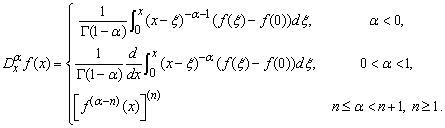(1)

Some properties for the proposed modified Riemann–Liouville derivative are listed in  as follows: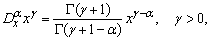(2)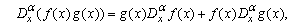(3)(4)

The above equations play an important role in fractional calculus in the following sections.

We present the main steps of the fractional sub-equation method as follows.

Setp1. Suppose that a nonlinear FDEs, say in two independent variables x and t, is given by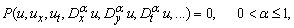(5)

where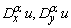and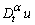are Jumarie’s modified Riemann–Liouville derivatives of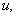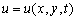is an unknown function,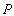is a polynomial in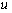and its various partial derivatives, in which the highest order derivatives and nonlinear terms are involved.

Setp2. By using the traveling wave transformation: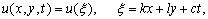(6)

where k, l and c are constants to be determined later, the FDE (5) is reduced to the following nonlinear fractional ordinary differential equation (ODE) for :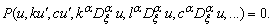(7)

Setp3. We suppose that Eq. (7) has the following solution: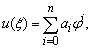(8)

whereare constants to be determined later, n is a positive integer determined by balancing the highest order derivatives and nonlinear terms in Eq. (5) or Eq. (7) (see Ref. for details), and satisfies the following fractional Riccati equation: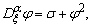(9)

where σ is a constant. By using the generalized Exp-function method via Mittag-Leffler functions , Zhang et al. first obtained the following solutions of fractional Riccati equation (9)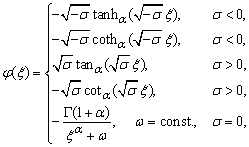(10)

where the generalized hyperbolic and trigonometric functions are defined as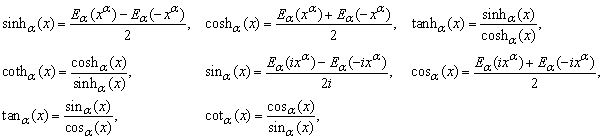where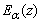denotes the Mittag-Leffler function, given as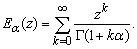Setp4. Substituting Eq. (8) along with Eq. (9) into Eq. (7) and using the properties of Jumarie’s modified Riemann–Liouville derivative (2)–(4), we can get a polynomial in . Setting all the coefficients of to zero, yields a set of overdetermined nonlinear algebraic equations for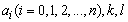and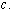Setp5. Assuming that the constants and c can be obtained by solving the algebraic equations in Step 4, substituting these constants and the solutions of Eq.(9) into Eq.(8), we can obtain the explicit solutions of Eq.(5) immediately.

Remark: If , the Riccati equation become used in . So the method in this example can be used to solve integer-order differential equations. In this sense, we would like to conclude that our method includes the existing tanh-function method as special case.

### 3. Applications of the Method

In this section, we apply the fractional sub-equation method to construct the exact analytical solutions for some nonlinear fractional PDEs, namely the space–time fractional PKP equation and the space–time fractional SRLW equation which are very important nonlinear fractional PDEs in mathematical physics and have been paid attention by many researchers.

3.1. Example 1. The Space–time Fractional PKP Equation

We first consider the space-time fractional PKP equation  in the form: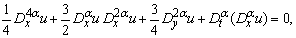(11)

By considering the traveling wave transformation Eq.(11) can be reduced to the following nonlinear fractional ODE: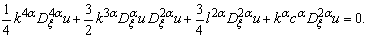(12)

By balancing the highest order derivative terms and nonlinear terms in Eq. (12), we suppose that Eq. (12 ) has the following formal solution:(13)

where satisfies Eq. (9).

Substituting Eq.(13) along with Eq.(9) into Eq.(12) and then setting the coefficients of to zero, we can obtain a set of algebraic equations for as follows:(14)

Solving the algebraic equations( 14 ) by Maple or Mathematica, we have:(15)

where is an arbitrary constant.

We, therefore, obtain from Eqs. (10), (13) and (15) three types of exact solutions of Eq.(11), namely, two generalized hyperbolic function solutions, two generalized trigonometric function solutions and one rational solution as follows: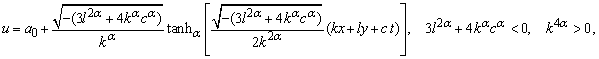(16)(17)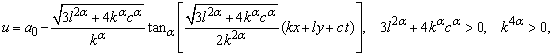(18)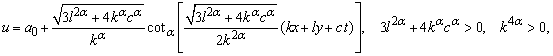(19)(20)

As , these obtained exact solutions give the ones of the standard form equation of the space-time fractional PKP equation (11).

3.2. Example 2. The Space-time Fractional SRLW Equation

We next consider the following space-time fractional SRLW equation(21)

which arises in severed physical applications including ion sound waves in plasma. Using the traveling wave transformation Eq.(21) can be reduced to the following nonlinear fractional ODE:(22)

According to the method described in Section 2, we suppose that Eq.(22) has the following formal solution: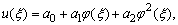(23)

where satisfies Eq. (9).

Substituting Eq.(23) along with Eq.(9) into Eq.(22) and collect the coefficients of and set them to be zero, a set of algebraic equations are obtained as follows: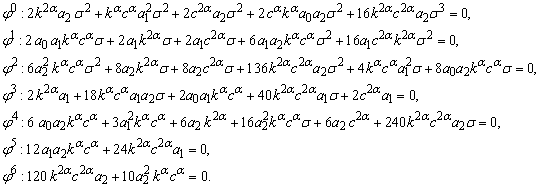(24)

Solving the set of algebraic equations( 24) by Maple or Mathematica yields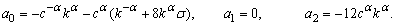(25)

Finally, from Eqs.(10), (23) and (25) we obtain the following generalized hyperbolic function solutions, generalized trigonometric function solutions and rational solution of Eq.(21)(26)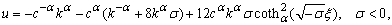(27)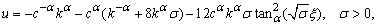(28)(29)(30)

where .

### 4. Conclusion

In this paper, we have seen that three types of exact analytical solutions including the generalized hyperbolic function solutions, generalized trigonometric function solutions and rational solutions for the space-time fractional KPK equation and the space-time fractional SRLW equation are successfully found out by using the fractional sub-equation method.

From our results obtained in this paper, we conclude that the fractional sub-equation method is powerful, effective and convenient for nonlinear fractional PDEs . Also, the solutions of the proposed nonlinear fractional PDEs in this paper have many potential applications in physics and engineering. Finally, this method provides a powerful mathematical tool to obtain more general exact analytical solutions of a great many nonlinear fractional PDEs in mathematical physics.

To the best of our knowledge, the solutions obtained in this paper have not been reported in literature.

### References

  A. Kilbas, H. M. Srivastava, and J. J. Trujillo, Theory and Applications of Fractional Differential Equations, vol. 204 of North-Holland Mathematics Studies, Elsevier Science, Amsterdam, The Netherlands,2006.In article  R. Hilfer, Applications of Fractional Calculus in Physics, World Scientific Publishing, River Edge, NJ,USA, 2000.In article CrossRef PubMed  B. J. West, M. Bologna, and P. Grigolini, Physics of Fractal Operators, Springer, New York, NY, USA, 2003.In article CrossRef  K. S. Miller and B. Ross, An Introduction to the Fractional Calculus and Fractional Differential Equations, John Wiley & Sons, New York, NY,USA, 1993.In article  S. G. Samko, A. A. Kilbas, and O. I. Marichev, Fractional Integrals and Derivatives, Gordon and Breach Science, Yverdon, Switzerland, 1993.In article  I. Podlubny, Fractional Differential Equations, vol. 198 of Mathematics in Science and Engineering,Academic Press, San Diego, Calif, USA, 1999.In article  K. B. Oldham and J. Spanier, The Fractional Calculus, Academic Press, New York, NY, USA, 1974.In article  V. Kiryakova, Generalized Fractional Calculus and Applications, vol. 301 of Pitman Research Notes in Mathematics Series, Longman Scientific & Technical, Harlow, UK, 1994.In article  I. Podlubny, Fractional Differential Equations, vol. 198 of Mathematics in Science and Engineering,Academic Press, New York, NY, USA, 1999.In article  J. Sabatier, O. P. Agrawal, and J. A. T. Machado, Advances in Fractional Calculus: Theoretical Developments and Applications in Physics and Engineering, Springer, New York, NY, USA, 2007.In article CrossRef  F. Mainardi, Fractional Calculus and Waves in Linear Viscoelasticity: An Introduction to Mathematical Models, Imperial College Press, London, UK, 2010.In article CrossRef  D. Baleanu, K. Diethelm, E. Scalas, and J. J. Trujillo, Fractional Calculus: Models and Numerical Methods, vol. 3 of Series on Complexity, Nonlinearity and Chaos, World Scientific Publishing, Boston, Mass, USA,2012.In article  X. J. Yang, Local Fractional Functional Analysis and Its Applications, Asian Academic Publisher, Hong Kong.In article  X. J. Yang, Advanced Local Fractional Calculus and Its Applications, World Science Publisher, New York, NY, USA, 2012.In article  A. H. A. Ali, The modified extended tanh-function method for solving coupled MKdV and coupled Hirota-Satsuma coupled KdV equations, Phys. Lett.A, 363(2007) 420.In article CrossRef  C. Li, A. Chen and J. Ye, Numerical approaches to fractional calculus and fractional ordinary differential equation, J. Comput .Phys, 230(2011) 3352.In article CrossRef  G. H. Gao, Z. Z. Sun and Y. N. Zhang, A finite difference scheme for fractional sub-diffusion equations on an unbounded domain using artificial boundary conditions, J. Comput .Phys, 231(2012) 2865.In article CrossRef  W. Deng, Finite element method for the space and time fractional Fokker-Planck equation, SIAM J . Numer. Anal, 47(2008/09) 204.In article CrossRef  S. Momani, Z. Odibat and V. S. Erturk, Generalized differential transform method for solving a space- and time-fractional diffusion-wave equation, Phys. Lett. A, 370(2007) 379.In article CrossRef  Z. Odibat and S. Momani, A generalized differential transform method for linear partial differential equations of fractional order, Appl. Math. Lett, 21(2008)194.In article CrossRef  Y. Hu, Y. Luo and Z. Lu, Analytical solution of the linear fractional differential equation by Adomian decomposition method, J. Comput. Appl. Math, 215(2008)220.In article CrossRef  A. M. A. El-Sayed and M. Gaber, The Adomian decomposition method for solving partial differential equations of fractal order in finite domains, Phys. Lett. A, 359(2006)175.In article CrossRef  A. M. A. El-Sayed, S. H. Behiry and W. E. Raslan, Adomian’s decomposition method for solving an intermediate fractional advection-dispersion equation, Comput . Math. Appl, 59(2010)1759.In article CrossRef  Z. Odibat and S. Momani, The variational iteration method: an efficient scheme for handling fractional partial differential equations in fluid mechanics, Comput . Math. Appl, 58(2009)2199.In article CrossRef  M. Inc, The approximate and exact solutions of the space- and time-fractional Burgers equations with initial conditions by variational iteration method, J. Math. Anal. Appl, 345(2008)476.In article CrossRef  G. C. Wu and E. W. M. Lee, Fractional variational iteration method and its application, Phys. Lett. A, 374(2010)2506.In article CrossRef  J.-H.He, Homotopy perturbation technique, Comput Methods. Appl. Mech. Eng, 178(1999)257.In article CrossRef  E. Fan, Soliton solutions for a generalized Hirota-Satsuma coupled KdV equation and a coupled MKdV equation, Phys. Lett. A, 282(2001)18.In article CrossRef  A. Saadatmandi and M. Dehghan, A new operational matrix for solving fractional- order diﬀerential equations, Comput. Math. Appl, 59 (2010) 1326.In article CrossRef  Y. Zhou, F. Jiao and J. Li, Existence and uniqueness for p-type fractional neutral diﬀerential equations, Nonlinear Anal, 71 (2009) 2724.In article CrossRef  S. Zhang and H. Q. Zhang, Fractional sub-equation method and its applications to nonlinear fractional PDEs, Phys. Lett. A, 375(2011)1069.In article CrossRef  M. L. Wang, Solitary wave solutions for variant Boussinesq equations,Phys. Lett. A, 199(1995)169.In article CrossRef  G. Jumarie, Modified Riemann-Liouville derivative and fractional Taylor series of nondifferentiable functions further results, Comput. Math. Appl, 51(2006)1367.In article CrossRef  G. Jumarie, Fractional partial differential equations and modified Riemann-Liouville derivative new methods for solution, J. Appl. Math. Comput, 24 (2007) 31.In article CrossRef  S. Guo, L. Mei, Y. Li and Y. Sun, The improved fractional sub-equation method and its applications to the space-time fractional differential equations in fluid mechanics, Phys. Lett. A, 376(2012) 407.In article CrossRef  B. Lu, Bä cklund transformation of fractional Riccati equation and its applications to nonlinear fractional partial differential equations, Phys. Lett. A, 376 (2012) 2045.In article CrossRef  Y. B. Zhou, M. L. Wang and Y. M. Wang, Periodic wave solutions to a coupled KdV equations with variable coefficients, Phys.Lett.A, 308(2003)31.In article CrossRef  S. Zhang, Q. A. Zong, D. Liu and Q. Gao, A generalized Exp-function method for fractional Riccati differential equations,Commun. Fract. Calc, 1 (2010) 48.In article  Z. S. Lü and H. Q. Zhang, On a new modiﬁed extended tanh-function method, Commun. Theor. Phys. (Beijing, China), 39(2003) 405.In article  A. Borhanifar and M.M. Kabir, New periodic and soliton solutions by application of Exp-function method for nonlinear evolution equations, J. Comput. Appl. Math, 229 (2009) 158.In article CrossRef  Fei .Xu, Application of Exp-function method to Symmetric Regularized Long Wave (SRLW) equation. Phys. Lett. A, 372 (2008) 252.In article CrossRef